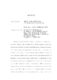## MEANS AND AVERAGING ON RIEMANNIAN MANIFOLDS2009
##### Authors
Afsari, Bijan
Processing of manifold-valued data has received considerable attention in recent years. Standard data processing methods are not adequate for such data. Among many related data processing tasks finding means or averages of manifold-valued data is a basic and important one. Although means on Riemannian manifolds have a long history, there are still many unanswered theoretical questions about them, some of which we try to answer. We focus on two classes of means: the Riemannian $L^{p}$ mean and the recursive-iterative means. The Riemannian $L^{p}$ mean is defined as the solution(s) of a minimization problem, while the recursive-iterative means are defined based on the notion of Mean-Invariance (MI) in a recursive and iterative process. We give a new existence and uniqueness result for the Riemannian $L^{p}$ mean. The significant consequence is that it shows the local and global definitions of the Riemannian $L^{p}$ mean coincide under an uncompromised condition which guarantees the uniqueness of the local mean. We also study smoothness, isometry compatibility, convexity and noise sensitivity properties of the $L^{p}$ mean. In particular, we argue that positive sectional curvature of a manifold can cause high sensitivity to noise for the $L^{2}$ mean which might lead to a non-averaging behavior of that mean. We show that the $L^{2}$ mean on a manifold of positive curvature can have an averaging property in a weak sense. We introduce the notion of MI, and study a large class of recursive-iterative means. MI means are related to an interesting class of dynamical systems that can find Riemannian convex combinations. A special class of the MI means called pairwise mean, which through an iterative scheme called Perimeter Shrinkage is related to cyclic pursuit on manifolds, is also studied. Finally, we derive results specific to the special orthogonal group and the Grassmannian manifold, as these manifolds appear naturally in many applications. We distinguish the $2$-norm Finsler balls of appropriate radius in these manifolds as domains for existence and uniqueness of the studied means. We also introduce some efficient numerical methods to perform the related calculations in the specified manifolds.